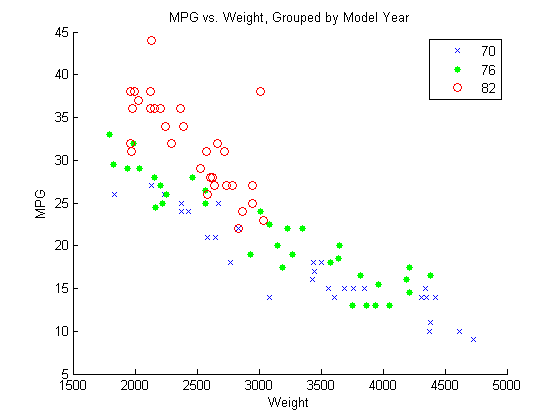## Linear Regression with Categorical Covariates

This example shows how to perform a regression with categorical covariates using categorical arrays and `fitlm`.

`load carsmall`

The variable `MPG` contains measurements on the miles per gallon of 100 sample cars. The model year of each car is in the variable `Model_Year`, and `Weight` contains the weight of each car.

### Plot grouped data.

Draw a scatter plot of `MPG` against `Weight`, grouped by model year.

```figure() gscatter(Weight,MPG,Model_Year,'bgr','x.o') title('MPG vs. Weight, Grouped by Model Year')```The grouping variable, `Model_Year`, has three unique values, `70`, `76`, and `82`, corresponding to model years 1970, 1976, and 1982.

### Create table and categorical array.

Create a table that contains the variables `MPG`, `Weight`, and `Model_Year`. Convert the variable `Model_Year` to a categorical array.

```cars = table(MPG,Weight,Model_Year); cars.Model_Year = categorical(cars.Model_Year);```

### Fit a regression model.

Fit a regression model using `fitlm` with `MPG` as the dependent variable, and `Weight` and `Model_Year` as the independent variables. Because `Model_Year` is a categorical covariate with three levels, it should enter the model as two indicator variables.

The scatter plot suggests that the slope of `MPG` against `Weight` might differ for each model year. To assess this, include weight-year interaction terms.

The proposed model is

`$E\left(MPG\right)={\beta }_{0}+{\beta }_{1}Weight+{\beta }_{2}I\left[1976\right]+{\beta }_{3}I\left[1982\right]+{\beta }_{4}Weight×I\left[1976\right]+{\beta }_{5}Weight×I\left[1982\right],$`

where I and I are dummy variables indicating the model years 1976 and 1982, respectively. I takes the value 1 if model year is 1976 and takes the value 0 if it is not. I takes the value 1 if model year is 1982 and takes the value 0 if it is not. In this model, 1970 is the reference year.

`fit = fitlm(cars,'MPG~Weight*Model_Year')`
```fit = Linear regression model: MPG ~ 1 + Weight*Model_Year Estimated Coefficients: Estimate SE ___________ __________ (Intercept) 37.399 2.1466 Weight -0.0058437 0.00061765 Model_Year_76 4.6903 2.8538 Model_Year_82 21.051 4.157 Weight:Model_Year_76 -0.00082009 0.00085468 Weight:Model_Year_82 -0.0050551 0.0015636 tStat pValue ________ __________ (Intercept) 17.423 2.8607e-30 Weight -9.4612 4.6077e-15 Model_Year_76 1.6435 0.10384 Model_Year_82 5.0641 2.2364e-06 Weight:Model_Year_76 -0.95953 0.33992 Weight:Model_Year_82 -3.2329 0.0017256 Number of observations: 94, Error degrees of freedom: 88 Root Mean Squared Error: 2.79 R-squared: 0.886, Adjusted R-Squared: 0.88 F-statistic vs. constant model: 137, p-value = 5.79e-40```

The regression output shows:

• `fitlm` recognizes `Model_Year` as a categorical variable, and constructs the required indicator (dummy) variables. By default, the first level, `70`, is the reference group (use `reordercats` to change the reference group).

• The model specification, `MPG~Weight*Model_Year`, specifies the first-order terms for `Weight` and `Model_Year`, and all interactions.

• The model R2 = 0.886, meaning the variation in miles per gallon is reduced by 88.6% when you consider weight, model year, and their interactions.

• The fitted model is

`$M\stackrel{^}{P}G=37.4-0.006Weight+4.7I\left[1976\right]+21.1I\left[1982\right]-0.0008Weight×I\left[1976\right]-0.005Weight×I\left[1982\right].$`

Thus, the estimated regression equations for the model years are as follows.

Model YearPredicted MPG Against Weight
1970

`$M\stackrel{^}{P}G=37.4-0.006Weight$`

1976

`$M\stackrel{^}{P}G=\left(37.4+4.7\right)-\left(0.006+0.0008\right)Weight$`

1982

`$M\stackrel{^}{P}G=\left(37.4+21.1\right)-\left(0.006+0.005\right)Weight$`

The relationship between `MPG` and `Weight` has an increasingly negative slope as the model year increases.

### Plot fitted regression lines.

Plot the data and fitted regression lines.

```w = linspace(min(Weight),max(Weight)); figure() gscatter(Weight,MPG,Model_Year,'bgr','x.o') line(w,feval(fit,w,'70'),'Color','b','LineWidth',2) line(w,feval(fit,w,'76'),'Color','g','LineWidth',2) line(w,feval(fit,w,'82'),'Color','r','LineWidth',2) title('Fitted Regression Lines by Model Year')```### Test for different slopes.

Test for significant differences between the slopes. This is equivalent to testing the hypothesis

`$\begin{array}{l}{H}_{0}:{\beta }_{4}={\beta }_{5}=0\\ {H}_{A}:\text{\hspace{0.17em}}{\beta }_{i}\ne 0\text{\hspace{0.17em}}\text{\hspace{0.17em}}\text{for}\text{\hspace{0.17em}}\text{\hspace{0.17em}}\text{at}\text{\hspace{0.17em}}\text{least}\text{\hspace{0.17em}}\text{\hspace{0.17em}}\text{one}\text{\hspace{0.17em}}\text{\hspace{0.17em}}i.\end{array}$`

`anova(fit)`
```ans = SumSq DF MeanSq F pValue Weight 2050.2 1 2050.2 263.87 3.2055e-28 Model_Year 807.69 2 403.84 51.976 1.2494e-15 Weight:Model_Year 81.219 2 40.609 5.2266 0.0071637 Error 683.74 88 7.7698 ```
This output shows that the p-value for the test is `0.0072` (from the interaction row, `Weight:Model_Year`), so the null hypothesis is rejected at the 0.05 significance level. The value of the test statistic is `5.2266`. The numerator degrees of freedom for the test is `2`, which is the number of coefficients in the null hypothesis.

There is sufficient evidence that the slopes are not equal for all three model years.